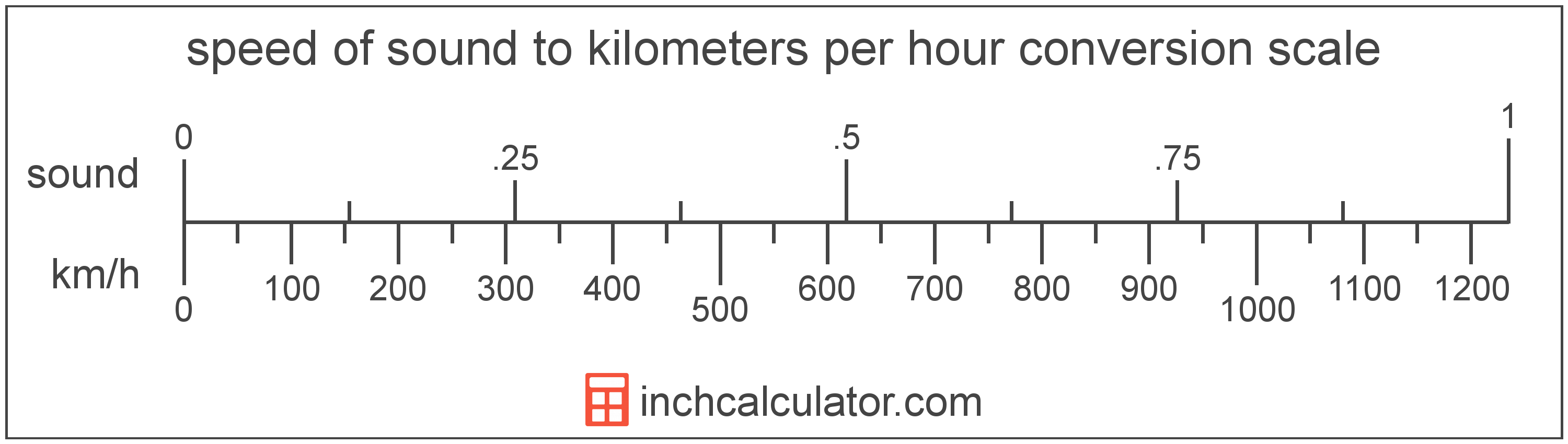# Kilometers per Hour to Speed of Sound Converter

Enter the speed in kilometers per hour below to get the value converted to speed of sound.

Results in Speed of Sound:1 km/h = 0.00081 sound

Do you want to convert speed of sound to kilometers per hour?

## How to Convert Kilometers per Hour to Speed of Sound

To convert a measurement in kilometers per hour to a measurement in speed of sound, multiply the speed by the following conversion ratio: 0.00081 speed of sound/kilometer per hour.

Since one kilometer per hour is equal to 0.00081 speed of sound, you can use this simple formula to convert:

speed of sound = kilometers per hour × 0.00081

The speed in speed of sound is equal to the speed in kilometers per hour multiplied by 0.00081.

For example, here's how to convert 5,000 kilometers per hour to speed of sound using the formula above.
speed of sound = (5,000 km/h × 0.00081) = 4.049239 soundKilometers per hour and speed of sound are both units used to measure speed. Keep reading to learn more about each unit of measure.

## What Are Kilometers per Hour?

Kilometers per hour are a measurement of speed expressing the distance traveled in kilometers in one hour.

The kilometer per hour, or kilometre per hour, is an SI unit of speed in the metric system. Kilometers per hour can be abbreviated as km/h, and are also sometimes abbreviated as kph. For example, 1 kilometer per hour can be written as 1 km/h or 1 kph.

In the expressions of units, the slash, or solidus (/), is used to express a change in one or more units relative to a change in one or more other units. For example, km/h is expressing a change in length or distance relative to a change in time.

Kilometers per hour can be expressed using the formula:
vkm/h = dkm / thr

The velocity in kilometers per hour is equal to the distance in kilometers divided by time in hours.

## What is the Speed of Sound?

The speed of sound is the distance a sound wave travels through an elastic medium. The speed of sound through air at 20 °C is equal to 343 meters per second, or roughly 767 miles per hour.

Speed of sound can be abbreviated as sound; for example, 1 speed of sound can be written as 1 sound.

## Kilometer per Hour to Speed of Sound Conversion Table

Table showing various kilometer per hour measurements converted to speed of sound.
Kilometers Per Hour Speed Of Sound
1 km/h 0.00081 sound
2 km/h 0.00162 sound
3 km/h 0.00243 sound
4 km/h 0.003239 sound
5 km/h 0.004049 sound
6 km/h 0.004859 sound
7 km/h 0.005669 sound
8 km/h 0.006479 sound
9 km/h 0.007289 sound
10 km/h 0.008098 sound
20 km/h 0.016197 sound
30 km/h 0.024295 sound
40 km/h 0.032394 sound
50 km/h 0.040492 sound
60 km/h 0.048591 sound
70 km/h 0.056689 sound
80 km/h 0.064788 sound
90 km/h 0.072886 sound
100 km/h 0.080985 sound
200 km/h 0.16197 sound
300 km/h 0.242954 sound
400 km/h 0.323939 sound
500 km/h 0.404924 sound
600 km/h 0.485909 sound
700 km/h 0.566893 sound
800 km/h 0.647878 sound
900 km/h 0.728863 sound
1,000 km/h 0.809848 sound
2,000 km/h 1.6197 sound
3,000 km/h 2.4295 sound
4,000 km/h 3.2394 sound
5,000 km/h 4.0492 sound
6,000 km/h 4.8591 sound
7,000 km/h 5.6689 sound
8,000 km/h 6.4788 sound
9,000 km/h 7.2886 sound
10,000 km/h 8.0985 sound

## References

1. National Institute of Standards and Technology, NIST Guide to the SI, Chapter 6: Rules and Style Conventions for Printing and Using Units, https://www.nist.gov/pml/special-publication-811/nist-guide-si-chapter-6-rules-and-style-conventions-printing-and-using
2. CK-12, Speed of Sound, https://www.ck12.org/physics/speed-of-sound/lesson/Speed-of-Sound-MS-PS/